﻿ 基于可靠性分析的立方星网络维护架构优化Download PDF文章快速检索 高级检索

1. 中国科学院 空间应用工程与技术中心, 北京 100094;
2. 中国科学院大学 计算机与控制学院, 北京 100049

Maintenance architecture optimization of CubeSat networks based on reliability analysis
FU Honglan1,2, ZHANG Hao1, GAO Yang1
1. Technology and Engineering Center for Space Utilization, Chinese Academy of Sciences, Beijing 100094, China;
2. School of Computer and Control Engineering, University of Chinese Academy of Sciences, Beijing 100049, China
Abstract: CubeSat formations or constellations can form distributed space sensor networks, enhancing the ability of the CubeSat to execute sophisticated space missions. However, CubeSats are prone to malfunction, and the uncertainty of their failure time causes the performance instability of the sensor network, highlighting the importance of on-orbit maintenance of CubeSat networks. In view of the function maintenance of space CubeSat sensor networks, a maintenance architecture is described. It can improve the rapid responsiveness and recovery capability to a single CubeSat fault event of the network by launching CubeSats regularly, making backup CubeSats on the orbit and replacing damaged CubeSats in time. The operation cost model of this architecture is built, involving fixed costs, storage costs and shortage costs. The practical CubeSat lifetime data is collected to acquire the optimal CubeSat lifetime stochastic model with the parameter estimation optimization method of maximizing the coefficient of determination. The supply time and quantity of spare CubeSats are optimized by a Monte-Carlo-simulation-based genetic algorithm. This solution is a trade-off between the costs of backups and the losses caused by the system performance decline, finally achieving an optimal comprehensive income.
Keywords: CubeSat failure data    space sensor networks    on-orbit maintenance architecture    on-orbit service    Weibull distribution

Gu等提出基于航空部件寿命分布的部件采购策略以处理部件需求的不确定性，并且假定部件寿命服从正态分布。Du Jonchay和Ho描述了一种由运载火箭、机器人服务设备和轨道仓库组成的机器人在轨服务基础设施，这篇文章假定故障发生服从泊松过程。然而航天器故障行为并不一定遵守正态分布或者泊松过程，真实的模型应该来自真实的数据。部分学者对小卫星数据进行统计分析，结果表明Weibull分布适合用于建模卫星以及卫星子系统的故障行为

1 维护架构与成本模型 1.1 维护架构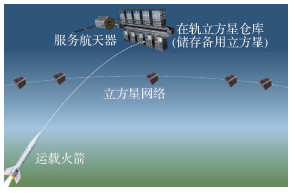图 1 立方星网络维护架构 Fig. 1 Maintenance architecture of CubeSat networks

1.2 成本模型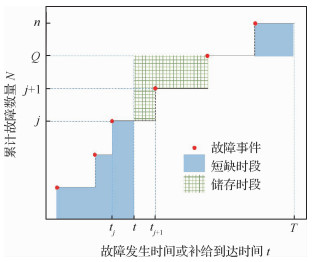图 2 成本计算示意图(j+1 < Q < n) Fig. 2 Cost calculation diagram (j+1 < Q < n)

 $\begin{array}{*{20}{l}} {M = s\left[ {\sum\limits_{k = 1}^j {(t - {t_k})} + \sum\limits_{k = Q + 1}^n {(T - {t_k})} } \right] + }\\ {{\kern 1pt} {\kern 1pt} {\kern 1pt} {\kern 1pt} {\kern 1pt} {\kern 1pt} {\kern 1pt} {\kern 1pt} {\kern 1pt} {\kern 1pt} {\kern 1pt} {\kern 1pt} {\kern 1pt} {\kern 1pt} {\kern 1pt} {\kern 1pt} {\kern 1pt} {\kern 1pt} {\kern 1pt} {\kern 1pt} {\kern 1pt} {\kern 1pt} {\kern 1pt} h\sum\limits_{k = j + 1}^Q {({t_k} - t)} + cQ} \end{array}$ （1）

 $\begin{array}{l} M = \\ \left\{ {\begin{array}{*{20}{l}} {s\left[ {\sum\limits_{k = 1}^Q {(t - {t_k})} + \sum\limits_{k = Q + 1}^n {(T - {t_k})} } \right] + cQ}&{Q \le j}\\ {s\left[ {\sum\limits_{k = 1}^Q {(t - {t_k})} + \sum\limits_{k = Q + 1}^n {(T - {t_k})} } \right] + cQ}&{}\\ {{\kern 1pt} {\kern 1pt} {\kern 1pt} {\kern 1pt} {\kern 1pt} {\kern 1pt} {\kern 1pt} {\kern 1pt} {\kern 1pt} {\kern 1pt} {\kern 1pt} {\kern 1pt} {\kern 1pt} {\kern 1pt} h\sum\limits_{k = j + 1}^{{\rm{min}}(Q,n)} {({t_k} - t)} + h(T - t) \cdot }&{}\\ {{\kern 1pt} {\kern 1pt} {\kern 1pt} {\kern 1pt} {\kern 1pt} {\kern 1pt} {\kern 1pt} {\kern 1pt} {\kern 1pt} {\kern 1pt} {\kern 1pt} {\kern 1pt} {\kern 1pt} [{\rm{max}}(Q,n) - n] + cQ}&{Q \ge j + 1} \end{array}} \right. \end{array}$ （2）
2 立方星可靠性模型

2.1 立方星寿命数据

 卫星编号NORAD ID 发射日期 立方星寿命或任务持续时间/天 删失标记 26065 2000/1/27 1 0 37250 2010/12/8 22 1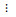43131 2018/1/12 67 0
2.2 非参数分析

Kaplan-Meier(KM)估计方法可以从包含删失数据的故障数据中推导出经验可靠性函数。可靠性函数又称为生存函数，对于立方星而言，其可靠性函数表示立方星故障发生在t时刻之后的概率，计算公式为

 $R(t) = \prod\limits_{i:{t_i} \le t} {\frac{{{n_i} - {a_i}}}{{{n_i}}}}$ （3）

2.3 参数分析

1) 最大化拟合优度参数估计方法

 ${{R^2} = 1 - \frac{{{S_{{\rm{ err }}}}}}{{{S_{{\rm{ tot }}}}}}}$ （4）
 ${{S_{{\rm{ err }}}} = \sum\limits_{i = 1}^n {(R(} {t_i}) - {R^\prime }({t_i},\alpha ){)^2}}$ （5）
 ${{S_{{\rm{ tot }}}} = \sum\limits_{i = 1}^n {(R(} {t_i}) - \bar R{)^2}}$ （6）
 ${\bar R = \frac{1}{n}\sum\limits_{i = 1}^n R ({t_i})}$ （7）

 ${\alpha ^*} = {\rm{arg}}{\kern 1pt} {\kern 1pt} {\rm{max}}{\kern 1pt} {\kern 1pt} {R^2}(\alpha )$ （8）

2) 对数正态分布

 $R(x) = \frac{1}{2} - {\rm{erf}} \left( {\frac{{{\rm{ln}}{\kern 1pt} {\kern 1pt} x - \mu }}{{\sigma \sqrt 2 }}} \right)$ （9）

3) Weibull分布

Weibull分布广泛应用于可靠性分析中，能灵活描述不同故障行为，其PDF和CDF分别为

 $\begin{array}{*{20}{l}} {f(x;\beta ,\eta ) = }\\ {{\kern 1pt} {\kern 1pt} {\kern 1pt} {\kern 1pt} {\kern 1pt} {\kern 1pt} {\kern 1pt} {\kern 1pt} {\kern 1pt} {\kern 1pt} {\kern 1pt} {\kern 1pt} {\kern 1pt} {\kern 1pt} {\kern 1pt} {\kern 1pt} {\kern 1pt} {\kern 1pt} {\kern 1pt} {\kern 1pt} {\kern 1pt} {\kern 1pt} \left\{ {\begin{array}{*{20}{l}} {\left( {\frac{\beta }{\eta }} \right){{\left( {\frac{x}{\eta }} \right)}^{(\beta - 1)}}{\rm{exp}}( - {{(x/\eta )}^\beta })}&{x \ge 0}\\ 0&{x < 0} \end{array}} \right.} \end{array}$ （10）
 $F(x;\beta ,\eta ) = 1 - {\rm{exp}}( - {(x/\eta )^\beta })$ （11）

 $R(x) = {\rm{exp}}( - {(x/\eta )^\beta })$ （12）
3 仿真方法

 $\begin{array}{*{20}{l}} {\mathop {{\rm{min}}}\limits_{t,Q} {\kern 1pt} {\kern 1pt} {\kern 1pt} {\kern 1pt} \frac{1}{N}\sum\limits_{l = 1}^N M (t,Q,s,h,c,{{\{ t\} }_l})}\\ {{\rm{s}}{\rm{.}}{\kern 1pt} {\kern 1pt} {\kern 1pt} {\kern 1pt} {\rm{t}}{\rm{.}}{\kern 1pt} {\kern 1pt} {\kern 1pt} {\kern 1pt} {\kern 1pt} {\kern 1pt} {\kern 1pt} {\kern 1pt} {\kern 1pt} \left\{ {\begin{array}{*{20}{l}} {t \in (1,T)}\\ {Q \in (1,{Q_m})} \end{array}} \right.} \end{array}$ （13）

4 结果分析 4.1 立方星寿命分布模型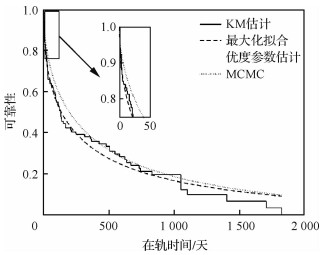图 3 不同参数估计方法对比 Fig. 3 Contrast of different parameter estimation methods

 方法 分布 参数 数值 R2 最大化拟合优度参数估计 对数 μ 4.785 6 0.977 2 正态 σ 2.445 6 Weibull η 291.900 4 0.986 3 MCMC Weibull β 0.476 8 η 385.532 1 0.967 4 β 0.546 7

 立方星 η β 早期立方星 253.451 5 0.474 3 近期立方星 414.687 5 0.453 2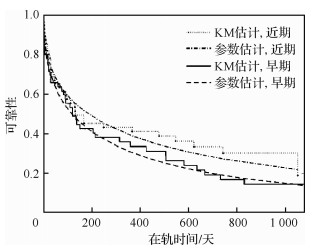图 4 早期、近期立方星对比 Fig. 4 Contrast of early and recent CubeSats
4.2 参数对总成本的影响

1) 单一成本情况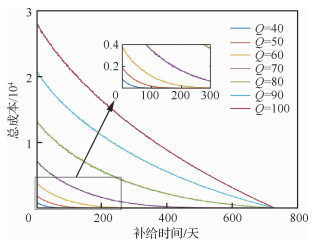图 5 储存成本对总成本的影响(c:h:s=0:1:0) Fig. 5 Effect of holding costs on total costs(c:h:s=0:1:0)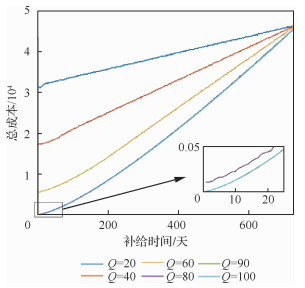图 6 短缺成本对总成本的影响(c:h:s=0:0:1) Fig. 6 Effect of shortage costs on total costs(c:h:s=0:0:1)

2) 全部成本情况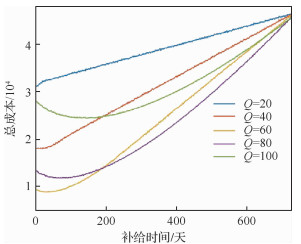图 7 3种成本参数对总成本的影响(c:h:s=1:1:1) Fig. 7 Effect of three cost parameters on total costs(c:h:s=1:1:1)

4.3 最优补给时刻与最优补给数量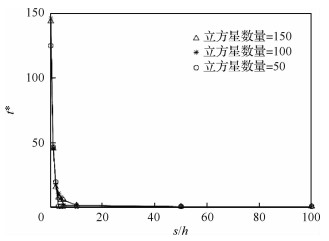图 8 s/h对t*的影响(不考虑固定成本) Fig. 8 Influence of s/h on t* (without considering fixed costs)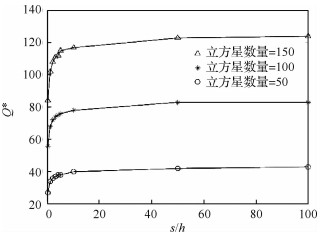图 9 s/h对Q*的影响(不考虑固定成本) Fig. 9 Influence of s/h on Q* (without considering fixed costs)
4.4 不同可靠性模型对应的成本比较

 模型 η β Nano 190 0.446 4 Micro 6 874 0.292 0

 模型 t* Q* 总成本 立方星 67 44 7 767.5 Nano 74 35 8 717.2 Micro 34 28 21 871.8
4.5 早期、近期立方星补给策略对比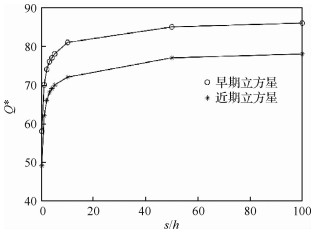图 10 早期、近期立方星Q*对比 Fig. 10 Q* comparison between early and recent CubeSats
5 结论

1) 立方星寿命分布的参数模型可采用Weibull分布或对数正态分布，进而采用最大化拟合优度参数估计方法进行参数估计，拟合数据表明Weibull模型精度比对数正态分布模型稍高。

2) 立方星具有早期失效率高的特点，由于这一特点，备用立方星最优补给时刻建议为规划周期早期。目前立方星总的特征寿命大约为292天，近期发射的立方星的可靠性高于早期发射的立方星。

3) 基于本文收集的立方星寿命数据，若在轨服务成本以短缺成本为主，那么建议备用立方星补给时间不晚于第150天，补给数量与立方星网络规模有关。若以储存成本为主，建议备用立方星补给数量约等于立方星网络中立方星总数量的60%，补给时刻不晚于第300天。补给时刻随短缺成本/储存成本增加而提前，与立方星网络规模无明显相关关系；补给数量随短缺成本/储存成本增加而增加。

4) 近期发射立方星的最优补给数量比早期立方星的少，对立方星网络的补给策略进行优化时，不仅需要选择合适的可靠性模型，还需要考虑由于技术发展而导致的立方星可靠性模型的变化。

  HEIDT H, PUIG-SUARI J, MOORE A, et al. CubeSat: A new generation of picosatellite for education and industry low-cost space experimentation[C]//14th Annual AIAA/USU Conference on Small Satellites. Reston: AIAA, 2000.  SHIROMA W A, MARTIN L K, AKAGI J M, et al. CubeSats:A bright future for nanosatellites[J]. Open Engineering, 2011, 1(1): 9-15. Click to display the text  CHIN A, COELHO R, NUGENT R, et al. CubeSat: The pico-satellite standard for research and education[C]//AIAA SPACE 2008 Conference & Exposition. Reston: AIAA, 2008.  ZHANG H, GURFIL P. Nanosatellite cluster keeping under thrust uncertainties[J]. Journal of Guidance, Control, and Dynamics, 2014, 37(5): 1406-1414. Click to display the text  POGHOSYAN A, GOLKAR A. CubeSat evolution:Analyzing CubeSat capabilities for conducting science missions[J]. Progress in Aerospace Sciences, 2017, 88: 59-83. Click to display the text  Planet Labs Inc. Planet imagery and archive[EB/OL].[2019-11-01]. https://www.planet.com/products/planet-imagery/.  PERAL E, TANELLI S, HADDAD Z, et al. Raincube: A proposed constellation of precipitation profiling radars in CubeSat[C]//2015 IEEE International Geoscience and Remote Sensing Symposium. Piscataway: IEEE Press, 2015: 1261-1264.  CHEN H R, LIU J K, LONG L, et al. Lunar far side positioning enabled by a CubeSat system deployed in an Earth-Moon halo orbit[J]. Advances in Space Research, 2019, 64(1): 28-41. Click to display the text  BANDYOPADHYAY S, FOUST R, SUBRAMANIAN G P, et al. Review of formation flying and constellation missions using nanosatellites[J]. Journal of Space and Rockets, 2016, 53(3): 567-578. Click to display the text  WEN C X, ZHANG H, GURFIL P. Orbit injection considerations for cluster flight of nanosatellites[J]. Journal of Spacecraft and Rockets, 2014, 52(1): 196-208. Click to display the text  VILLELA T, COSTA C A, BRANDÃO A M, et al. Towards the thousandth CubeSat:A statistical overview[J]. International Journal of Aerospace Engineering, 2019(3): 1-13. Click to display the text  RIESING K. Orbit determination from two line element sets of ISS-deployed cubesats[C]//29th Annual AIAA/USU Conference on Small Satellites. Reston: AIAA, 2015.  项军华, 张育林. 基于卫星可靠度和MTTR星座空间备份策略设计[J]. 系统工程与电子技术, 2007, 29(9): 1576-1580. XIANG J H, ZHANG Y L. Design of spatial backup strategy for constellation based on satellite reliability and MTTR[J]. Systems Engineering and Electronics, 2007, 29(9): 1576-1580. (in Chinese) Cited By in Cnki (11) | Click to display the text  DU JONCHAY T S, HO K. Impact evaluation of an orbital depot on on-orbit servicing infrastructures dedicated to modularized earth-orbiting platforms[J]. Acta Astronautica, 2017, 132: 192-203. Click to display the text  胡敏, 宋旭民, 杨雪榕. 基于Petri网的Walker导航星座备份策略研究[J]. 航天器工程, 2017, 26(2): 14-21. HU M, SONG X M, YANG X R. Research on spare stratege of Walker navigation constellation based on Petri net[J]. Spacecraft Engineering, 2017, 26(2): 14-21. (in Chinese) Cited By in Cnki | Click to display the text  ZHANG H, MENG D B, ZONG Y Y, et al. A modeling and analysis strategy of constellation availability using on-orbit and ground added launch backup and its application in the reliability design for a remote sensing satellite[J]. Advances in Mechanical Engineering, 2018, 10(4): 1-6. Click to display the text  GU J Y, ZHANG G Q, LI K W. Efficient aircraft spare parts inventory management under demand uncertainty[J]. Journal of Air Transport Management, 2015, 42: 101-109. Click to display the text  CASTET J F, SALEH J H. Satellite and satellite subsystems reliability:Statistical data analysis and modeling[J]. Reliability Engineering & System Safety, 2009, 94(11): 1718-1728. Click to display the text  GUO J, MONAS L, GILL E. Statistical analysis and modelling of small satellite reliability[J]. Acta Astronautica, 2014, 98(1): 97-110. Click to display the text  CASTET J F, SALEH J H. Satellite reliability:Statistical data analysis and modeling[J]. Journal of Spacecraft and Rockets, 2009, 46(5): 1065-1076. Click to display the text  CROWDER M J, KIMBER A C, SMITH R L, et al. Statistical analysis of reliability data[M]. London: Routledge, 2017: 16-27.  ERIK K. Nanosats database[EB/OL].[2018-11-01]. https://www.nanosats.eu/.
http://dx.doi.org/10.7527/S1000-6893.2020.23696

0

#### 文章信息

FU Honglan, ZHANG Hao, GAO Yang

Maintenance architecture optimization of CubeSat networks based on reliability analysis

Acta Aeronautica et Astronautica Sinica, 2020, 41(7): 323696.
http://dx.doi.org/10.7527/S1000-6893.2020.23696